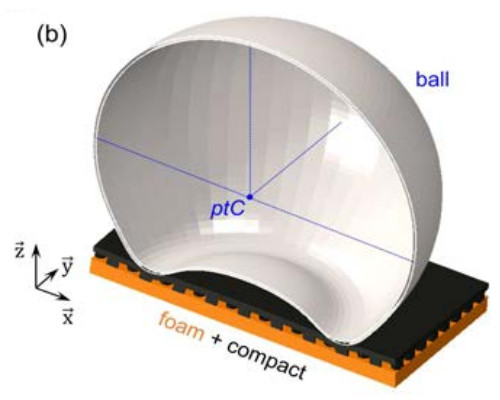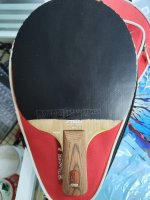# I saw a thread about the weight of impact. It should be force of impact.

#### brokenball

This user has no status.

#### brokenball

This user has no status.
##### Well-Known Member
Assuming a coefficient of restitution of 90% (blade with rubbers) and a 4 ms contact time.
For the velocity of the ball after impact we get:
cor = 90%, m_racket = 0.18 kg, m_ball = 0.0027 kg, v_racket_0 = 0 m/s, v_ball_0 = 10 m/s, m_total = 0.1827 kg

v_ball_1 = (m_ball * v_ball_0 + m_racket * v_racket_0 + m_racket * cor * (v_racket_0 - v_ball_0)) / m_total = -8.719 m/s

v_racket_1 = (m_ball * v_ball_0 + m_racket * v_racket_0 + m_ball * cor * (v_ball_0 - v_racket_0)) / m_total = 0.2807 m/s

For the average force we get: F_average t = m (v_ball_0 - v_ball_1) so
F_average = (0.0027 kg * (10 m/s - (-8.719 m/s))) / 0.004 s = 12.63 N
or for the racket F_average = (0.18 kg * (0 m/s - 0.2807 m/s)) / 0.004 s = -12.63 N
So if the impact speed is 10 m/s and the dwell/contact time is 4 ms. Lets assume that the ball is taking 2 ms to decelerate to a stop relative to the paddle. How far does the ball travel relative to the paddle in that time?

As usual there are some that want to distract the thread.

#### latej

This user has no status.

#### latej

This user has no status.
##### Active Member
alright.
there is where I say, no engrish
Let's spice the pop-corn up, Tony#### Attachments

• Non_Linearity_of_the_BallRubber_Impact_in_Table_Te.pdf
310.5 KB · Views: 26

#### ttarc

This user has no status.

#### ttarc

This user has no status.
##### Member
Let's spice the pop-corn up, TonyRinaldi and Manin are also coauthors of the newer paper "Dynamical buckling of a table-tennis ball impinging normally on a rigid target: experimental and numerical studies", Rémond et al., 2022
Now we only need to do some measurements on real rubbers and then we can try to calculate "How far does the ball travel relative to the paddle in that time?" or we simulate first, measure, tune the models used and maybe come up with an answer...

•latej

#### perkerk

This user has no status.

#### perkerk

This user has no status.
##### Member
I suspect that the relevant contribution by the dwell time on the paddle is just a variation/extension of the overall interaction of the paddle/ball collision itself.

There are various places advising how to apply force or power when hitting a ball for maximum effect, e.g.:

This site gives a good summary of elastic collisions: http://hyperphysics.phy-astr.gsu.edu/hbase/colsta.html

The case for a paddle and table tennis ball is the m1 >> m2 case where the projectile (paddle+arm) mass is much greater than the target (ball). There's also a calculator there if you want to see how negligible the effect is because of the difference in masses. The resultant velocity of the ball in the opposite direction when hitting approaches the paddle velocity + the incoming ball velocity. This would be in a perfect elastic collision, which of course it isn't, but it also makes it obvious that a stiffer paddle (i.e., creating closer to a perfect elastic collision) would also increase the resultant velocity of the ball.

#### brokenball

This user has no status.

#### brokenball

This user has no status.
##### Well-Known Member
In a purely elastic collision, when the projectile mass is much larger than the target, the resultant velocity of the target/ball is only a function of the incoming projectile/paddle and the target/ball velocities and nothing else.
OK, but the collision isn't purely elastic. There is the coefficient of restitution.

If you're trying to literally apply force for a duration through the shot, or to follow through the ball as you hit it, then you're not optimizing your swing to maximize the velocity at impact and instead wasting energy.
How do you apply force through the shot. Force is only applied to the ball during contact. You apply force to the paddle during the swing.

You want to use the above techniques not for how to hit the ball but instead to maximize the velocity of your paddle at impact.
Provided that is what you want to do when you make a flat kill, but not touch shots.

When I started paying attention to the velocity I could generate in the swing of my paddle, just based on the sound of it moving through the air, I quickly discovered that there was tension in my arm because I was trying apply a lot of force.

Instead, by relaxing my entire movement from core to arm to wrist and accelerating through the whole duration, I was able to create a much faster paddle velocity at impact and as a result could hit the ball harder.
You aren't clear, the duration of the stroke or duration of contact. It makes a difference.

This site gives a good summary of elastic collisions: http://hyperphysics.phy-astr.gsu.edu/hbase/colsta.html
That is a very good website but collisions aren't purely elastic. The formula in the linked web pages site do not include the the coefficient of restitution.

The case for a paddle and table tennis ball is the m1 >> m2 case where the projectile (paddle+arm) mass is much greater than the target (ball). There's also a calculator there if you want to see how negligible the effect is because of the difference in masses. The resultant velocity of the ball in the opposite direction when hitting approaches the paddle velocity + the incoming ball velocity. This would be in a perfect elastic collision, which of course it isn't, but it also makes it obvious that a stiffer paddle (i.e., creating closer to a perfect elastic collision) would also increase the resultant velocity of the ball.
Good, so you know the collision is not perfectly elastic.
This webpage is better.
Notice the formula for normal ( 3D perpendicular ) impulse.
Now assuming you know the actual contact time, one can calculate the average force needed to achieve that impulse. Which gets back to knowing the force of impact. However, this doesn't give a complete picture because when the ball makes initial contact the force is 0 and it increases until the ball is stopped relative to the paddle so the peak force is much higher than the average.

#### perkerk

This user has no status.

#### perkerk

This user has no status.
##### Member
Should have been obvious, and maybe to most, but to clarify this is for understanding the primary contributing factors affecting energy imparted into the incoming ball, and to understand the prevailing dynamic of a paddle/ball collision (in table tennis if also not obvious). I stand by what I said. It would appear you are taking exception to precisely how I worded this, and I would normally try to clarify for someone trying to understand this, but it honestly doesn't seem you are

Replies
20
Views
718Replies
2
Views
351
Replies
27
Views
2K
Replies
14
Views
2K
Replies
19
Views
2K